# GTCURV

Utility/Data Access SubroutineEvaluate a B-spline or user-written curve created with the Reference_ParamCurve entity.

## Use

The function can be called by any user-defined subroutine.

## Format

Fortran Calling Syntax
CALL GTCURV (id, alpha, iord, array, istat)
C/C++ Calling Syntax
c_gtcurv(id, alpha, iord, array, istat)
Python Calling Syntax
[array, istat] = py_gtcrv(id, alpha, iord)
MATLAB Calling Syntax
[array, istat] = m_gtcrv(id, alpha, iord)

## Attributes

ID
[integer]
The identifier of the corresponding Reference_ParamCurve.
ALPHA
[double precision]
The independent parameter alpha at which the curve is to be evaluated. For B-splines, alpha must satisfy -1 <= alpha <= 1.

For curves defined using CURSUB, alpha must satisfy MINPAR <= alpha <= MAXPAR.

IORD
[integer]
The variable that specifies the order of the derivative returned by GTCURV as follows:
Iord
Meaning
0
Returns the curve coordinate.
1
Returns the first derivative with respect to alpha.
2
Returns the second derivative with respect to alpha.

## Output

ARRAY
[double precision]
The array of three elements as shown below:
Iord Array First Element Array Second Element Array Third Element
0 $\text{x}$ $\text{y}$ $\text{z}$
1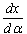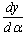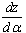2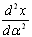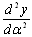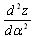All elements are evaluated at alpha.
ISTAT
[integer]
Variable that indicates the success or the reason for the failure of GTCURV.